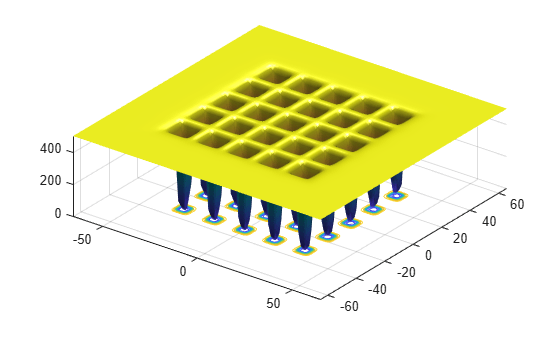# Optimize Function Using `particleswarm`, Problem-Based

This example shows how to minimize a function using particle swarm in the problem-based approach when the objective is a function file, possibly of unknown content (a "black box" function). The function to minimize, `dejong5fcn(x)`, is included when you run this example.

`dejong5fcn`Create a 2-D optimization variable `x`. The `dejong5fcn` function expects the variable to be a row vector, so specify `x` as a 2-element row vector.

`x = optimvar("x",1,2);`

To use `dejong5fcn` as the objective function, convert the function to an optimization expression using `fcn2optimexpr`.

`fun = fcn2optimexpr(@dejong5fcn,x);`

Create an optimization problem with the objective function `fun`.

`prob = optimproblem("Objective",fun);`

Set variable bounds from –50 to 50 in all components. When you specify scalar bounds, the software expands the bounds to all variables.

```x.LowerBound = -50; x.UpperBound = 50;```

Solve the problem, specifying the `particleswarm` solver.

```rng default % For reproducibility [sol,fval] = solve(prob,"Solver","particleswarm")```
```Solving problem using particleswarm. Optimization ended: relative change in the objective value over the last OPTIONS.MaxStallIterations iterations is less than OPTIONS.FunctionTolerance. ```
```sol = struct with fields: x: [-31.9751 -31.9719] ```
```fval = 0.9980 ```